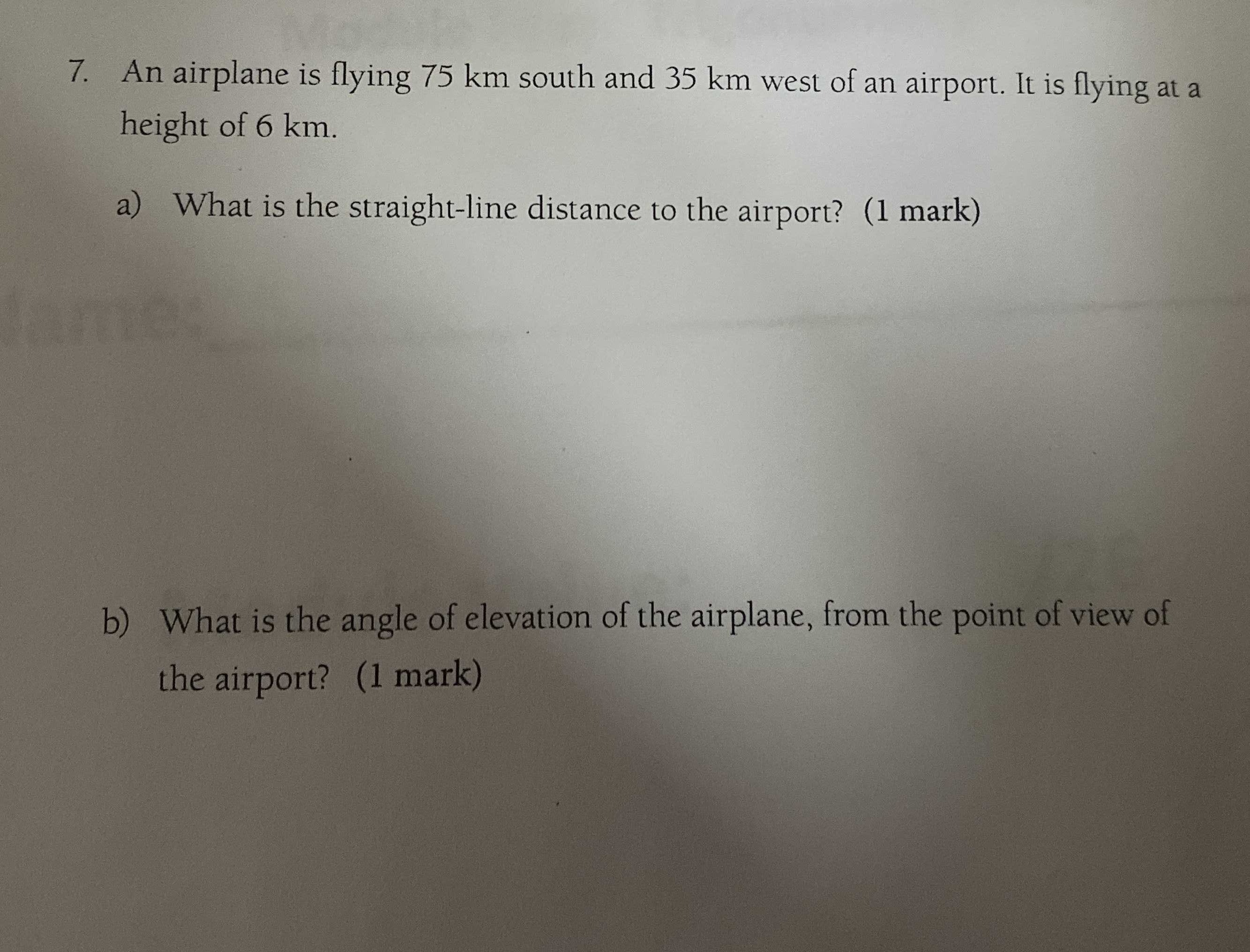### Still have math questions?

Trigonometry
QuestionAn airplane is flying $$75 km$$ south and $$35 km$$ west of an airport height of $$6 km$$ .

a) What is the straight-line distance to the airport?

b) What is the angle of elevation of the airplane, from the point airport?

b) angle of elevation = $$4.147°$$